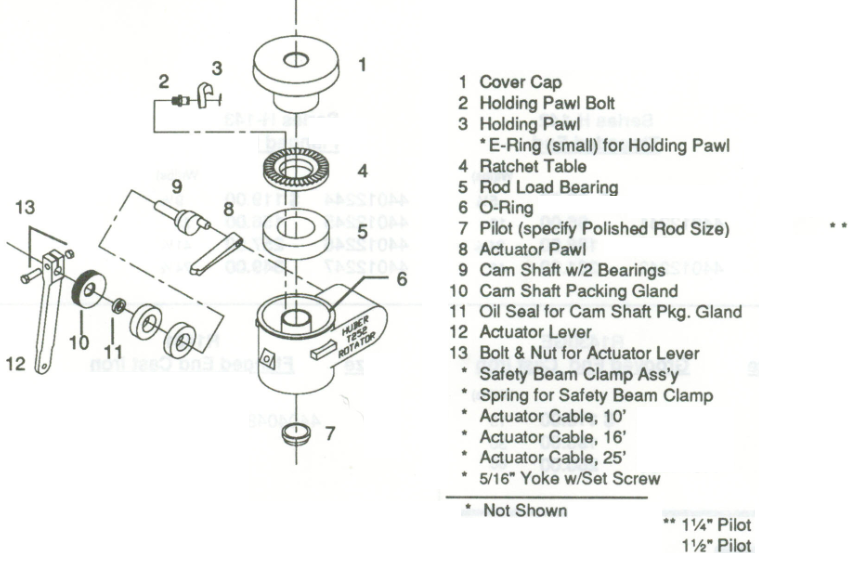Pressure loss calculations through a conduit

Whenever a fluid flows through a conduit pressure loss occurs. Many methods are available to calculate frictional pressure losses. They range from simple empirical equations to rigorous mechanistic multiphase flow models.

Darcy-Weisbach flow equation:

The Darcy-Weisbach flow equation is theoretically sound equation derived from the Conservation of Mass and Conservation of Momentum laws. Named after Henry Darcy and Julius Weisbach, it relates the pressure loss due to friction along a given length of pipe to the average velocity of the fluid flow for an incompressible fluid.

The Darcy-Weisbach equation contains a dimensionless friction factor, known as the Darcy friction factor. This is also variously called the Darcy–Weisbach friction factor, friction factor, resistance coefficient, flow coefficient, or Moody friction factor.

In a cylindrical pipe of uniform hydraulic diameter d, flowing full, the pressure loss due to density and viscous effects dp/dL is proportional to length L and can be characterized by the Darcy–Weisbach equation: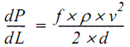Where:

• dP/dL = Pressure Gradient (psi/ft)
• f = friction factor
• ρ = Fluid density (lb/ft3)
• v = Fluid velocity (ft/s))
• d = Hydraulic diameter (ft))

The equation can be written as shown below in both typical US oilfield units and SI units:

This article will present the typical ranges of jet pump operating parameters and discusses the advantages and disadvantages of jet pumps in oil wells applications.

Jet Pump Operating Envelope: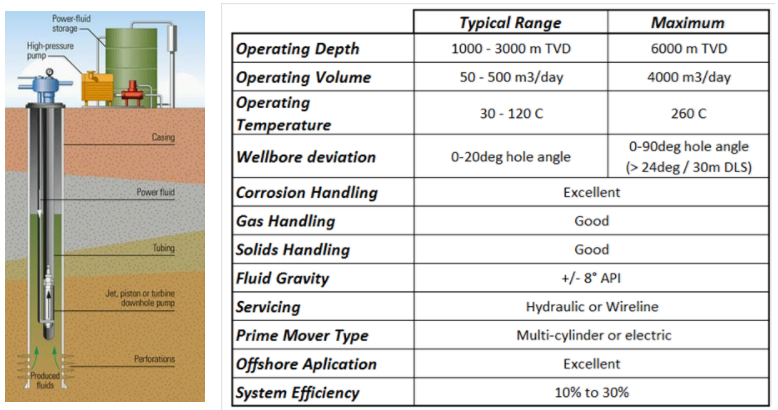Jet pumps have several advantages such as:

How Does Jet Pump Work?

The jet pump operates by pumping power fluid at high pressure and rate from surface to activate/drive a downhole pump. Looking at the downhole jet pump, there are three main components:

• Nozzle,
• Throat,
• And Diffuser.

The nozzle and throat are the key components of a jet pump. The ratio of the areas of these two parts is known as the area ratio of the pump and it determines the performance characteristics of the pump. Pumps with the same area ratio have the same performance and efficiency curves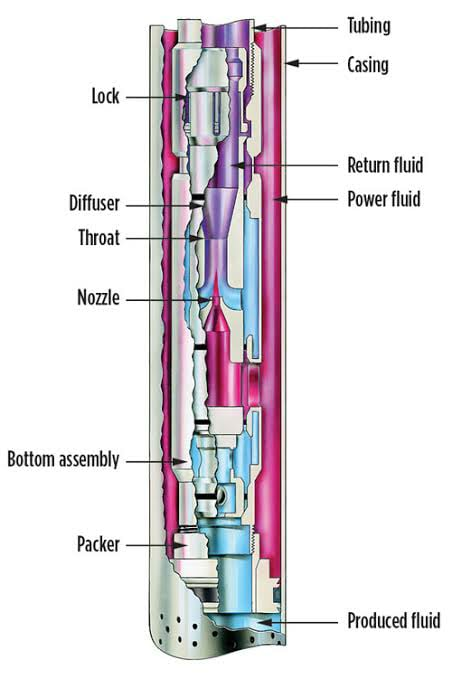Jet Pumps operates on Venturi principle. Above the JP, before entering the nozzle, the power fluid has high pressure (designated as PN) and low flow velocity.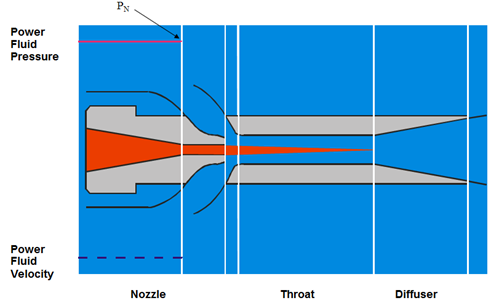While the power fluid passes the nozzle, due to the decrease in flow area it is transformed from low velocity, high static pressure flow to a high velocity, low static pressure flow (PS). This creates a pressure drop below the nozzle which drives the reservoir fluids into the pump.

Jet Pumps Working Principle

The first consumer of water jets pump was James Thompson back in 1852. In 1870, J. M. Rankin introduced the theory of jet pump operation, afterward, a number of papers have been published to develop the technology. The work of Gasoline and O’Brien in 1933 is considered to be the standard reference work. It has been based on both theoretical and experimental results. Since that time, developments in the technology have continued to the point that today jet pumps have high operational flexibility.

What is Hydraulic Lift?

As a general description, Hydraulic Lift (Jet Pumps) represents pumping power fluid at high pressure and rate from surface to activate/drive a downhole pump. Power Fluid can be water or oil.

With Jet Pump applications the completion needs to have minimum 3 flowing conduits:

1. A conduit for power fluid injection (inside of the tubing in case of standard flow, and in the annular space in case of reverse flow).
2. For reservoir fluid flow (below the JP & packer).
3. And a conduit for commingled fluid flow to the surface.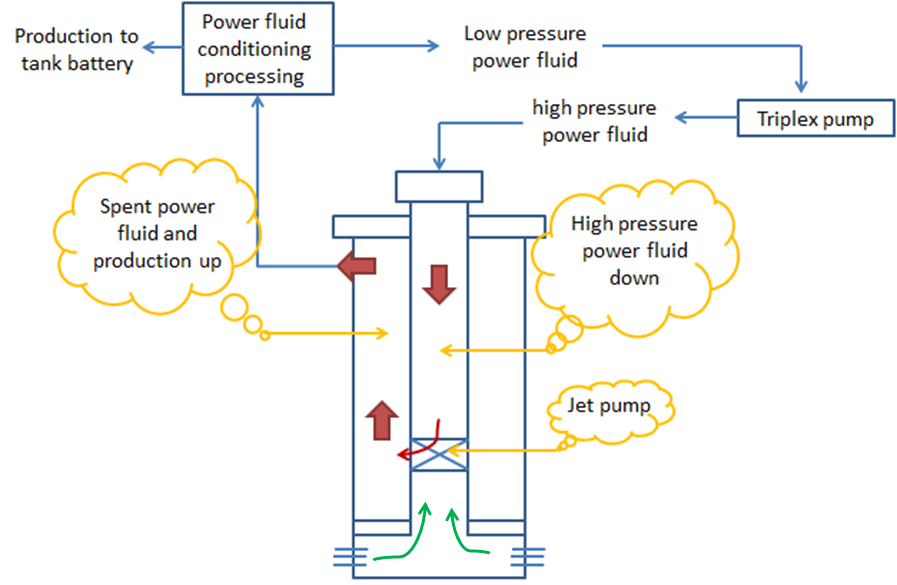Jet Pump working principle:

The jet pump artificial lift system is composed of two principal parts: the surface pumping equipment and the downhole jet pump. In the surface, the reciprocating pump transfers energy to the fluid increases its pressure, drove through surface piping, production tubing (or annular space) until the jet pump, placed on the bottom.

Rod Rotator extends the life of rod-pumped wells

The constant up-and-down movement of a sucker rod creates excessive friction between rods and tubing which can result in premature rod and tubing failures due to excessive wear. If left unchecked, this generally requires a costly intervention to make repairs.

To extend the sucker rod run life, one of the widely used techniques is the use of rod rotators. A rod rotator is a mechanical device installed on the polished rod between the carrier and the polished rod clamp. It incrementally rotates the rod with each stroke. A rotating mechanism with an actuator lever arm is connected to the walking beam with a metal string. As the surface unit moves up and down, it pulls and releases this metal string so that, moves the actuator lever arm up and down. The rotation mechanism is activated and this slowly rotates the polished rod and the rod string below.